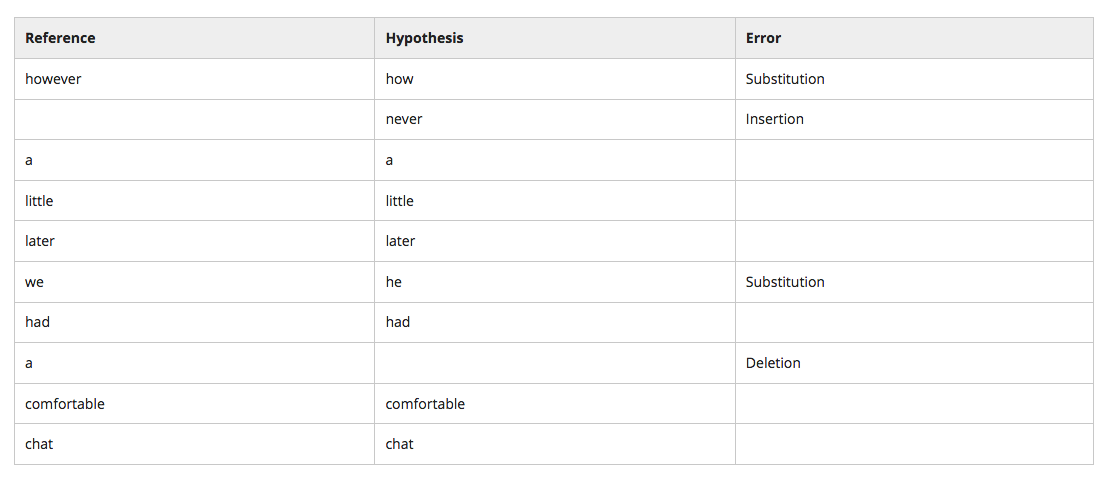# Automatic Speech Recognition (ASR Part 0)

Automatic Speech Recognition (ASR) systems are used for transcribing spoken text into words/sentences. ASR systems are complex systems consisting of multiple components, working in tandem to transcribe. In this blog series, I will be exploring the different components of a generic ASR system (although I will be using Kaldi for some references).

Any ASR system consists of the following basic components:

### Abbreviations

• LVCSR - Large Vocabulary Continuous Speech Recognition
• HMM - Hidden Markov Models
• AM - Acoustic Model
• LM - Language Model

### Data Requirements

The following are the data requirements for any ASR system

• Labeled Corpus: Collection of speech audio files and their transcriptions
• Lexicon: Mapping from the word to the series of phones to describe how the word is pronounced. Not necessary for phonetically written languages like Kannada.
• Data for training Language Model: Large text corpus (to train a statistical language model) in case we are looking to train an ASR system to handle generic/natural language inputs. If we are looking at constrained ASR systems that are only capable of transcribing a certain set of grammar rules (like PAN numbers, telephone numbers etc), we can directly write the grammar rules without training a statistical LM and hence this requirement is flexible.

### Bayes Rule in ASR

Any ASR follows the following principle.

$P(S|audio) = \frac{P(audio|S)P(S)}{P(audio)}$

Here, $$P(S)$$ is the LM and $$S$$ is the sentence.

$$P(audio)$$ is irrelevant since we are taking argmax. $$P(audio|S)$$ is the Acoustic Model. This describes distribution over the acoustic observations $$audio$$ given the word sequence $$S$$.

This equation is called as the Fundamental Equation of Speech Recognition

### Evaluation

Word Error Rate - $$WER = \frac{N_{sub} + N_{del} + N_{ins}}{N_{\text{reference_sentence}}}$$### Significance Testing

Statistical significance testing involves measuring to what degree the difference between two experiments (or algorithms) can be attributed to actual differences in the two algorithms or are merely the result inherent variability in the data, experimental setup or other factors.

Matched Pairs Testing